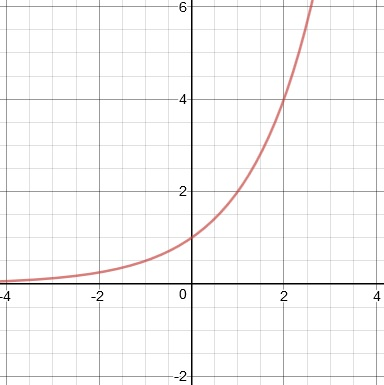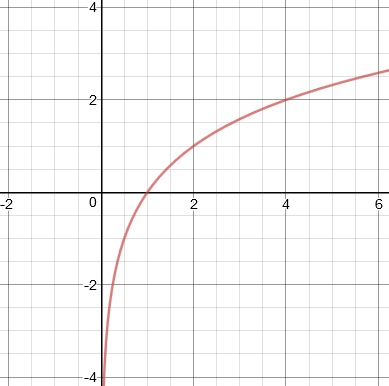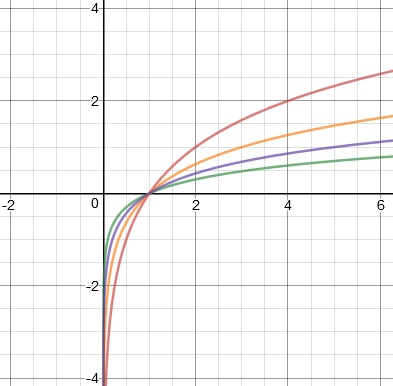Logarithmic Functions

Logarithmic Functions

Logarithmic functions, or logs, are the inverse of exponential functions.  The two can be written in either form.

y = bx     can be rewritten as    logb(y) = x

The main thing to remember about log functions is that they produce exponents.

EXAMPLES

Evaluate log2 32.

The question here is basically, "What exponent of 2 will give you 32?"  Remembering that 25 = 32, it follows that log2 32 = 5.

Evaluate log3 9.

Don't be fooled here.  This is not a division problem; the question is asking for an exponent.  Since 32 = 9, this means log3 9 = 2.

Evaluate log5 (1/25).

Remembering that negative exponents make reciprocals, we find that 5-2 = 1/25.  So log5 (1/25) = -2.

Evaluate log7 (-49).

There is no such exponent that satisfies 7? = -49.  Therefore, log7 (-49) does not exist.

Using the definition of logarithm, you can also solve equations.

EXAMPLE

Solve:    log2(x) = 4

Understand:

This reads "the log base two of a number equals four."  Solve by converting the logarithmic statement into its equivalent exponential form.

Solution:

log2(x) = 4

24 = x

16 = x

You can also get the graph of a log function from the graph of an exponential function.

Recall how y = 2x looks on the graph:The domain is all real numbers, the range is only the positive numbers, there's a y-intercept at 1, and there's a horizontal asymptote through y = 0.

If we think about reflecting this graph over the diagonal line y = x, we get the graph of y = log2(x).Notice with this function, the domain is only the positive numbers (remember how log7 (-49) didn't exist?), the range is all real numbers, there's an x-intercept at 1, and there's a vertical asymptote through x = 0.

Like with exponential functions, the appearance of the log function graph varies depending on the base, but all log graphs have the same general shape:y = log2 (x)

y = log3 (x)

y = log5 (x)

y = log10 (x)

The base 10 logarithm is also known as the common logarithm.  If the base isn't written, it's understood to be 10.

log (x) = log10 (x)